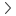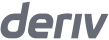# Stop loss and take profit calculator for multipliers

Traders' toolsPnL for multipliers

Level

Amount

0
0

Up

Down

0
0

Up

Down

### How to calculate stop loss and take profit level

The stop loss and take profit level for a contract on DTrader is calculated based on the formula:

Stop loss and take profit level in the Up direction = asset price × {(stop loss OR take profit amount + commission) ÷ (stake × multiplier) + 1}

Stop loss and take profit level in the Down direction = asset price × {(-stop loss OR take profit amount - commission) ÷ (stake × multiplier) + 1}

This helps you to set the stop loss and take profit level when the asset price moves in Up or Down direction.

### Example calculation

Let’s say you want to calculate the stop loss level when you open a position for Volatility 100 Index priced at 3376.24 USD with a stake amount of 10 USD, a multiplier value of x100, and a stop loss amount of 7.54 USD in Up direction.

3376.24
Asset price
x
{ ( -7.54
Stop loss or take profit amount
+
0.2 )
Commission 1
÷
( 10
Stake
x
100 )
Multiplier
+
1 }
=
3351.46
Stop loss level
3376.24
Asset price
x
{ ( -7.54
profit amount
Stop loss or take
+
0.2 )
Commission 1
÷
( 10
Stake
x
100 )
Multiplier
+
1 }
=
3351.46
Stop loss level
3376.24
Asset price
x
{ ( -7.54
Stop loss or take profit amount
+
0.2 )
Commission 1
÷
( 10
Stake
x
100 )
Multiplier
+
1 }
=
3351.46
Stop loss level
3376.24
Asset price
x
{ ( -7.54
profit amount
Stop loss or take
+
0.2 )
Commission 1
÷
( 10
Stake
x
100 )
Multiplier
+
1 }
=
3351.46
Stop loss level
1. The commission is automatically calculated based on your stake, symbol, and the multiplier you choose. Its value is directly available on DTrader.

So in this scenario, you would set your stop loss level at 3351.46.

Let’s say you want to calculate the take profit level when you open a position for Volatility 100 Index priced at 3376.24 USD with a stake amount of 10 USD, a multiplier value of x100, and a take profit amount of 7.54 USD in Down direction.

3376.24
Asset price
x
{ ( -7.54
Stop loss or take profit amount
-
0.2 )
Commission 1
÷
( 10
Stake
x
100 )
Multiplier
+
1 }
=
3350.11
Take profit level
3376.24
Asset price
x
{ ( -7.54
profit amount
Stop loss or take
-
0.2 )
Commission 1
÷
( 10
Stake
x
100 )
Multiplier
+
1 }
=
3350.11
Take profit level
3376.24
Asset price
x
{ ( -7.54
Stop loss or take profit amount
-
0.2 )
Commission 1
÷
( 10
Stake
x
100 )
Multiplier
+
1 }
=
3350.11
Take profit level
3376.24
Asset price
x
{ ( -7.54
profit amount
Stop loss or take
-
0.2 )
Commission 1
÷
( 10
Stake
x
100 )
Multiplier
+
1 }
=
3350.11
Take profit level
1. The commission is automatically calculated based on your stake, symbol, and the multiplier you choose. Its value is directly available on DTrader.

So in this scenario, you would set your take profit level at 3350.11.